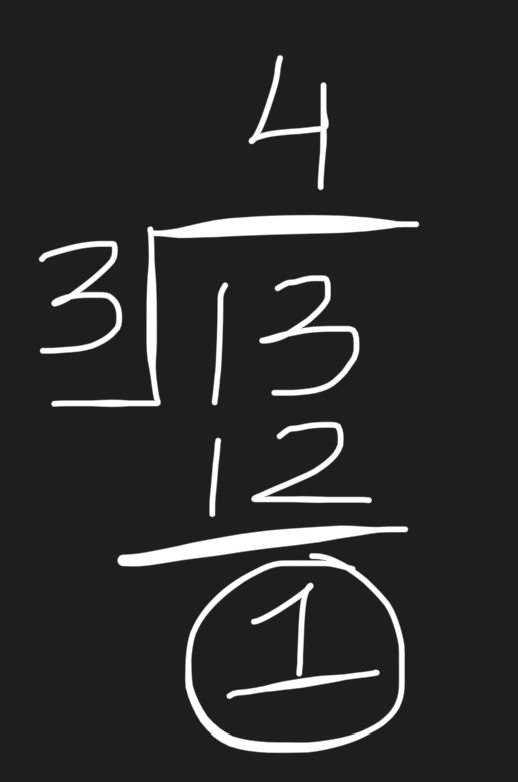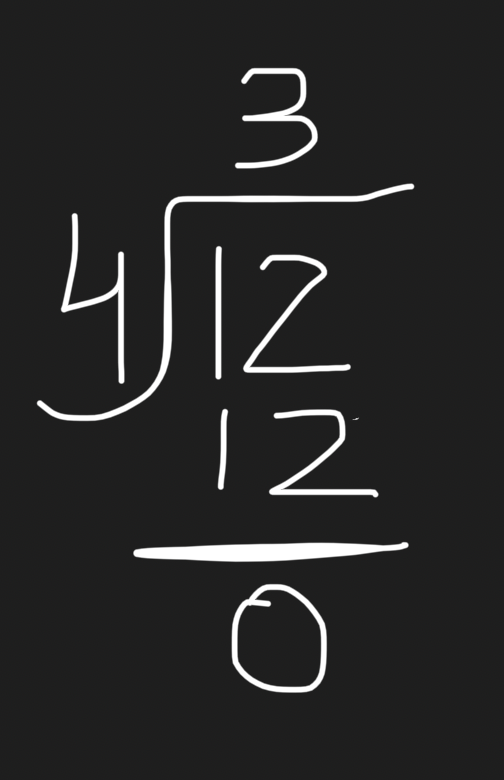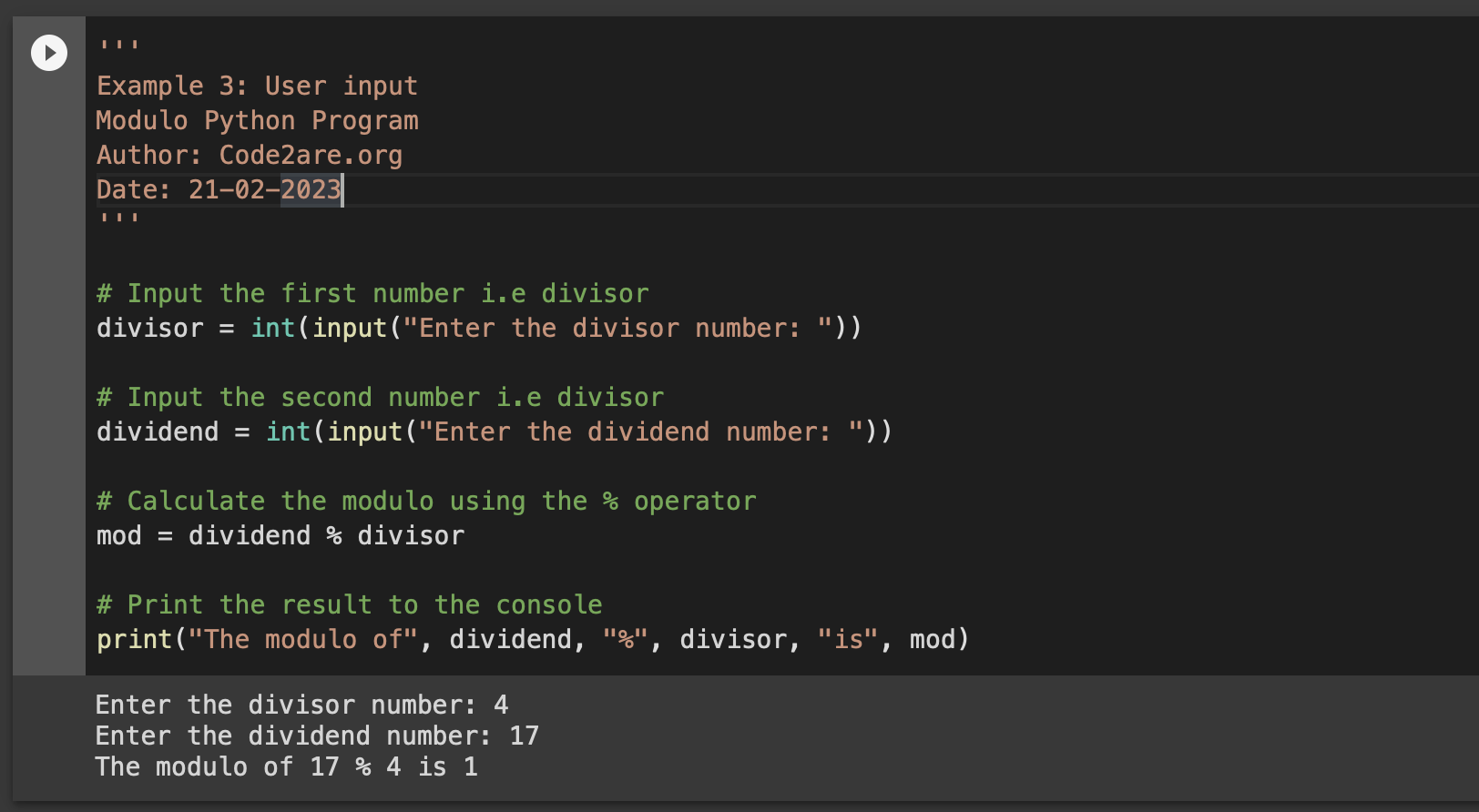# Program 10: Modulo of Two Numbers - 1000+ Python Programs

``````Question 10)

10.1) Write a program in Python to find the modulo of two numbers.
10.2) Python program to find the 10%2 (modulus)
10.3) Write a program to find the modulus of two numbers by taking user input using Python Programming.``````

## What is modulo operation?

The modulo operation in mathematics also known as "modulus" or "mod," is used to find the remainder that results when one number is divided by another.

% is the sign used to represent the modulo operator in mathematics as well as in Python Programming.

Modulo operator takes two operands:

• The Dividend - The number being divided.
• The Divisor - The number that the dividend is being divided by

The result of the modulo operation is the remainder left over after dividing the dividend by the divisor.

## Examples of modulo operation

Example 1: Let us perform the operation 13 % 3

The result is 1 because 13 divided by 3 will be 4 with a reminder of 1 as shown in the below working.Example 2: Let us perform the operation 12 % 4

The result is 0 because 12 divided by 4 will be 3 with a reminder of 0 as 4 is completely divisible by 4, see the below working.## Program 1: Write a program in Python to find the modulo of two numbers

``````'''
Example 1:
Modulo Python Program
Author: Code2are.org
Date: 21-02-2023
'''

# Let's select a divisor as int number 3
divisor = 3

# Let's select a dividend as int number 13
dividend = 13

#Calculate mod
mod = dividend % divisor

print(f"{dividend} % {divisor} = {mod}")``````
Output:

13 % 3 = 1

``````'''
Example 2:
Modulo Python Program
Author: Code2are.org
Date: 21-02-2023
'''

divisor = 2
dividend = 10

mod = dividend % divisor

print(f"{dividend} % {divisor} = {mod}")``````
Output:

10 % 2 = 0

## Write a program to find the modulus of two numbers by taking user input using Python Programming.

``````'''
Example 3: User input
Modulo Python Program
Author: Code2are.org
Date: 21-02-2023
'''

# Input the first number i.e divisor
divisor = int(input("Enter the divisor number: "))

# Input the second number i.e divisor
dividend = int(input("Enter the dividend number: "))

# Calculate the modulo using the % operator
mod = dividend % divisor

# Print the result to the console
print("The modulo of", dividend, "%", divisor, "is", mod)``````
Output:

Enter the divisor number: 4
Enter the dividend number: 17
The modulo of 17 % 4 is 1We have made use of the following Python functions:

1. print(): Built-in function to print a string on the console with f-strings formatting.
2. input(): Built-in function to accept user input

## References:

-

Have Questions? Post them here!